Ch 5. Rigid Body General Motion Multimedia Engineering Dynamics Fixed Axis Rotation Plane Motion Velocities Zero Velocity Point Plane Motion Accelerations Multiple Gears Rot. Coord. Velocities Rot. Coord. Acceleration
 Chapter - Particle - 1. General Motion 2. Force & Accel. 3. Energy 4. Momentum - Rigid Body - 5. General Motion 6. Force & Accel. 7. Energy 8. Momentum 9. 3-D Motion 10. Vibrations Appendix Basic Math Units Basic Equations Sections Search eBooks Dynamics Fluids Math Mechanics Statics Thermodynamics Author(s): Kurt Gramoll ©Kurt GramollDYNAMICS - CASE STUDY SOLUTION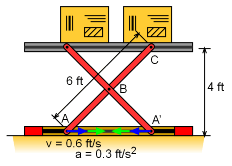Problem Diagram The velocity needs to be determined before the acceleration can be calculated. The motion of the lift can be determined using the following conditions; Point B only moves vertically Vertical speed of point C is twice that of point B Point C and the lift have the same vertical speed Velocity of Point B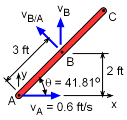Velocity Diagram for Member AC The geometric constraints of points A and B are critical in determining the member AC angular velocity. The velocity vectors for points A and B are      vA = vAi      vB = vBj The relative velocity equation for bar AB is      vB = vA + vB/A      vB = vA + ωAB × rAB      vBj = vAi + ωABk × rAB (cosθi + sinθj)           = vAi + ωAB rAB (cosθj - sinθi) Sum in both the i and j directions gives,      j :    vB = ωABrABcosθ      i :    vA = ωABrABsinθ There are only two unknowns, vA and ωAB, which can be solved for, giving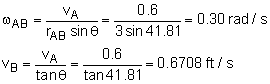The vertical velocities of points C and B are related by a factor of 2, giving,      vC = 2vB = 1.33 ft/s Acceleration of Point C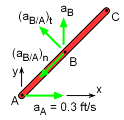Acceleration of Member AC As with the velocity, the geometric constraints of points A and B are used to determine their acceleration direction,      aA = aAi      aB = aBj The relative acceleration equation for bar AB is      aB = aA + aB/A          = aA + (aB/A)n + (aB/A)t          = aA + ωAB × (ωAB × rAB) + (αAB × rAB)      aBj = aAi + ωABk × (ωAB k × rAB (cosθi + sinθj))                + αABk × rAB (cosθi + sinθj)      aBj = aAi + ω2AB rAB (-cosθi - sinθj))               + αAB rAB (cosθj - sinθi) Summing in both the i and j directions gives,      i :    0 = aA - αABrABsinθ - ω2ABrABcosθ      j :    aB = αAB rAB cosθ - ω2ABrABsinθ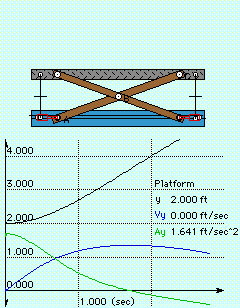Motion of Platform These two equations can be used to solve for the two unknowns, αAB and aB. Solving for αAB in the first equation and then substituting into the second equation gives,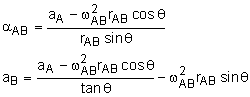Using numerical values give,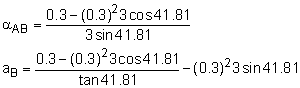αAB = 0.04938 rad/s2      aB = -0.06959 ft/s2 The vertical acceleration of the lift is twice the vertical acceleration of point B,      alift = 2aB = -0.1392 ft/s2

Practice Homework and Test problems now available in the 'Eng Dynamics' mobile app
Includes over 400 problems with complete detailed solutions.
Available now at the Google Play Store and Apple App Store.